#### 相似文章

26 十月 2021, 16:030
1 241

### 我们来看看这是为什么以及如何应对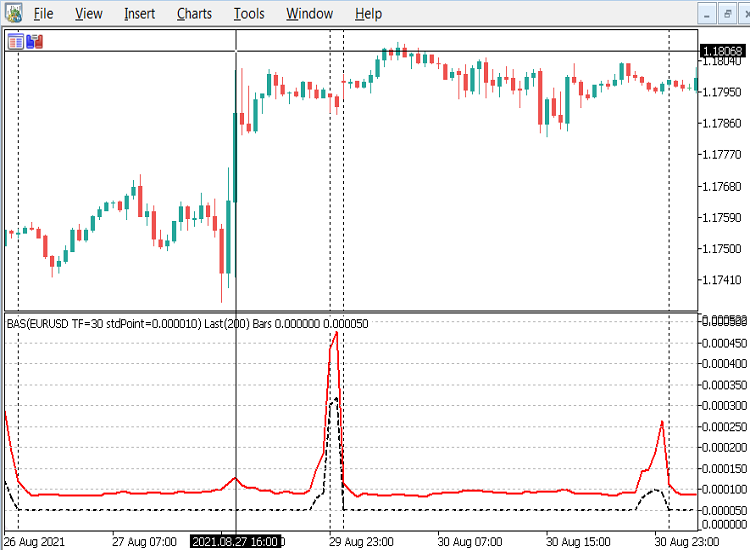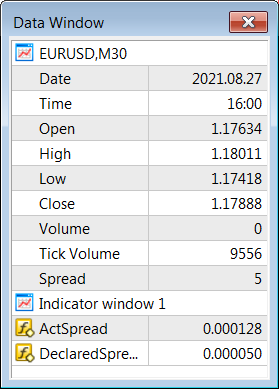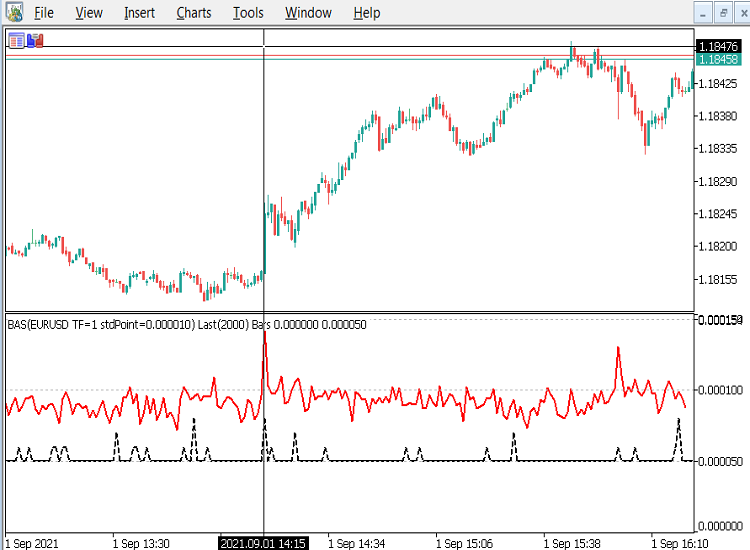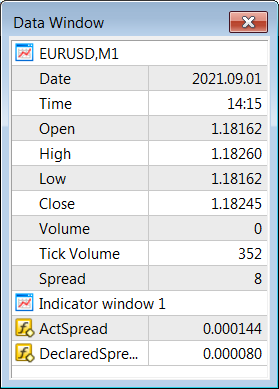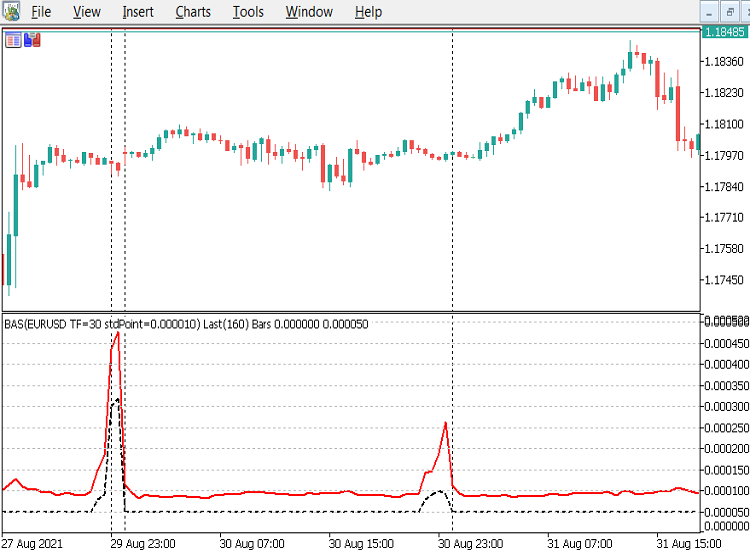### OnInit() 代码示例

```#property indicator_separate_window

#property indicator_buffers 2
#property indicator_plots   2

//--- plots
#property indicator_type1   DRAW_LINE
#property indicator_color1  clrRed
#property indicator_style1  STYLE_SOLID
#property indicator_width1  2

#property indicator_type2   DRAW_LINE
#property indicator_color2  clrBlack
#property indicator_style2  STYLE_DASH
#property indicator_width2  2

//--- indicator parameters
input int      numRecentBarsBack=100; //#RecentBarsBack M30+~100, M5~200, M1~500
input bool     doPrint=true;          //true=prints to the toolbox\experts log

//--- indicator buffers

//+------------------------------------------------------------------+
//| Custom indicator initialization function                         |
//+------------------------------------------------------------------+
int OnInit()
{
int numBars=iBars(_Symbol,PERIOD_CURRENT)-2;

// Check we have enough data for the request before we begin
if(numRecentBarsBack>numBars)
{
Alert("Can't Do ", numRecentBarsBack, "! Only ",
numBars, " Bars are Available",
" try 100 or so for 30+ minute charts,",
" 200 for 5 minute, or 500 for 1 minute charts.",
" Otherwise the indicator may be too slow"
);

return(INIT_PARAMETERS_INCORRECT);
}

double sumPrice=0;
double avgPrice=0;

// Get the standard 5 point spread for the standard EURUSD currency
double stdSpread=0.00005/iClose("EURUSD",PERIOD_M1,1); // 1.2 ~=  EURUSD std price

//Find out the current average price of the instrument we are using, so we can standardise the spread and _Point
int CheckAvgPriceBars=MathMin(numRecentBarsBack, 200);

int i=0;
for(; i<CheckAvgPriceBars; i++)
{
sumPrice+=iClose(_Symbol,PERIOD_CURRENT,i);
}
avgPrice=sumPrice/(i? i: 1.0);

//convert the stdSpread to stdPoint by dividing by 5, so we compare  apples with apples, not oranges

Print(i, "=bars done, avgPrice=", DoubleToString(avgPrice,6),
" stdPoint=", DoubleToString(stdPoint, 6)
);

string indName ="BAS("+_Symbol;
indName+=" TF="+string(_Period);
indName+=" stdPoint="+DoubleToString(stdPoint, 6);
indName+=") Last("+string(numRecentBarsBack)+") Bars";

IndicatorSetString(INDICATOR_SHORTNAME, indName);

IndicatorSetInteger(INDICATOR_DIGITS,6);

IndicatorSetDouble(INDICATOR_MINIMUM, 0.0);

IndicatorSetInteger(INDICATOR_LEVELS, 20);

//mark out each standard EURUSD 5 point spread, to compare this currencies spread with EURUSD
IndicatorSetDouble(INDICATOR_LEVELVALUE,0,  0.000000);
IndicatorSetDouble(INDICATOR_LEVELVALUE,1,  5*stdPoint);
IndicatorSetDouble(INDICATOR_LEVELVALUE,2, 10*stdPoint);
IndicatorSetDouble(INDICATOR_LEVELVALUE,3, 15*stdPoint);
IndicatorSetDouble(INDICATOR_LEVELVALUE,4, 20*stdPoint);
IndicatorSetDouble(INDICATOR_LEVELVALUE,5, 25*stdPoint);
IndicatorSetDouble(INDICATOR_LEVELVALUE,6, 30*stdPoint);
IndicatorSetDouble(INDICATOR_LEVELVALUE,7, 35*stdPoint);
IndicatorSetDouble(INDICATOR_LEVELVALUE,8, 40*stdPoint);
IndicatorSetDouble(INDICATOR_LEVELVALUE,9, 45*stdPoint);
IndicatorSetDouble(INDICATOR_LEVELVALUE,10,50*stdPoint);
IndicatorSetDouble(INDICATOR_LEVELVALUE,11,55*stdPoint);
IndicatorSetDouble(INDICATOR_LEVELVALUE,12,60*stdPoint);
IndicatorSetDouble(INDICATOR_LEVELVALUE,13,65*stdPoint);
IndicatorSetDouble(INDICATOR_LEVELVALUE,14,70*stdPoint);
IndicatorSetDouble(INDICATOR_LEVELVALUE,15,75*stdPoint);
IndicatorSetDouble(INDICATOR_LEVELVALUE,16,80*stdPoint);
IndicatorSetDouble(INDICATOR_LEVELVALUE,17,85*stdPoint);
IndicatorSetDouble(INDICATOR_LEVELVALUE,18,90*stdPoint);
IndicatorSetDouble(INDICATOR_LEVELVALUE,19,95*stdPoint);

return(INIT_SUCCEEDED);
}
```

OnInit() 的其余部分是相当标准的，除了指标子窗口中使用的水平，这些水平设置为对应于标准 EURUSD 5 点差的倍数的值。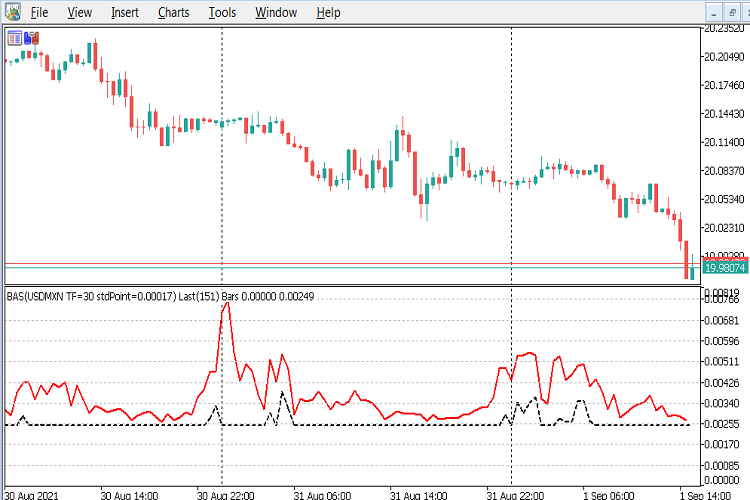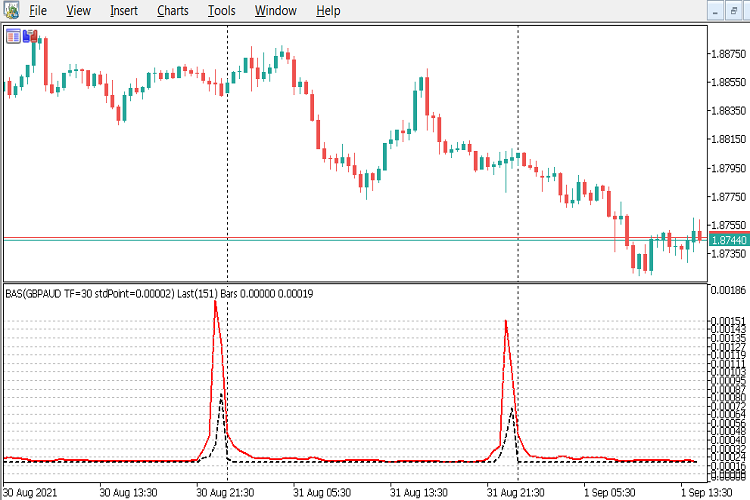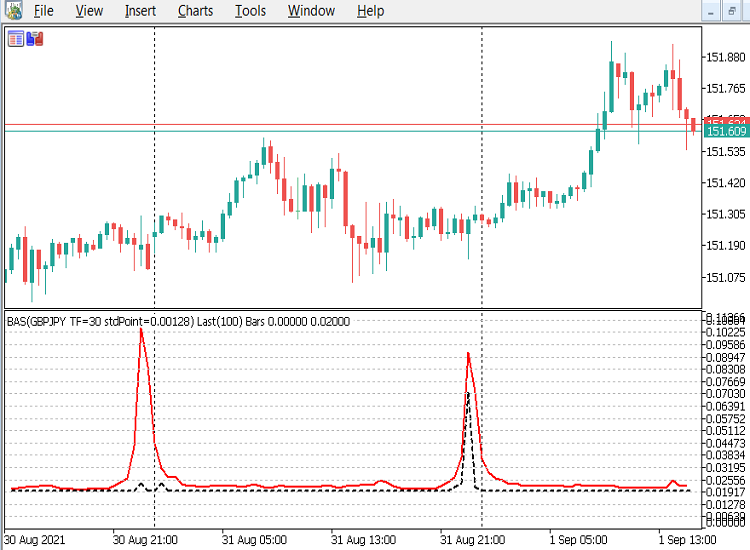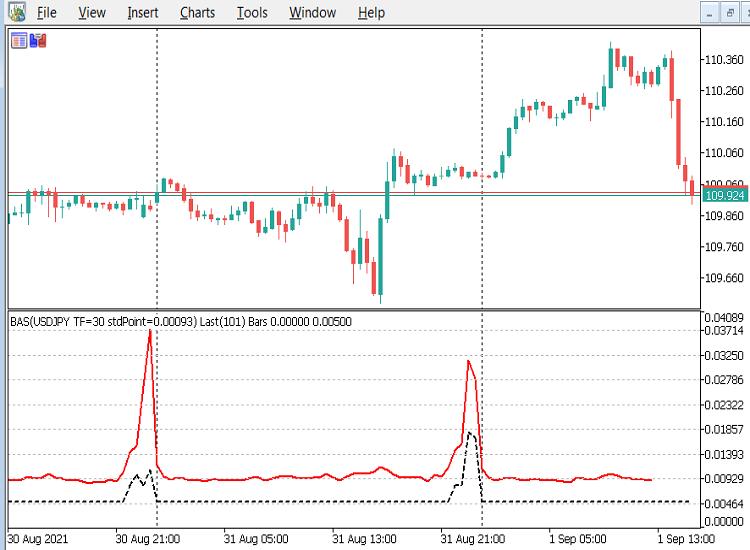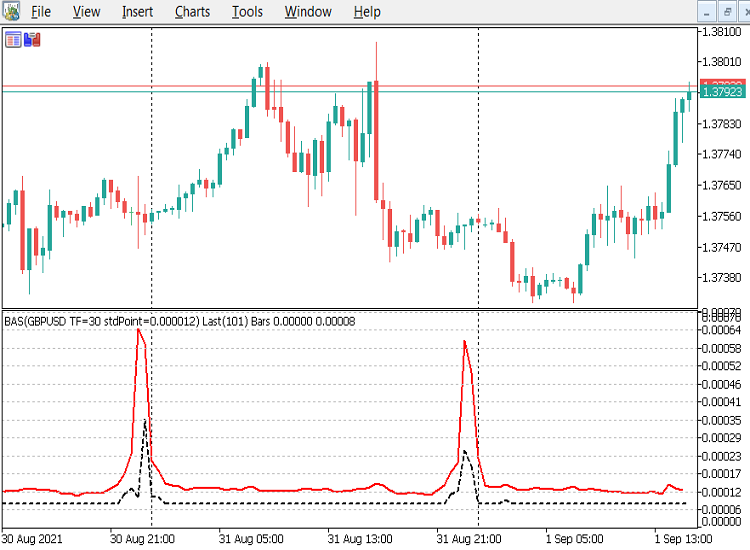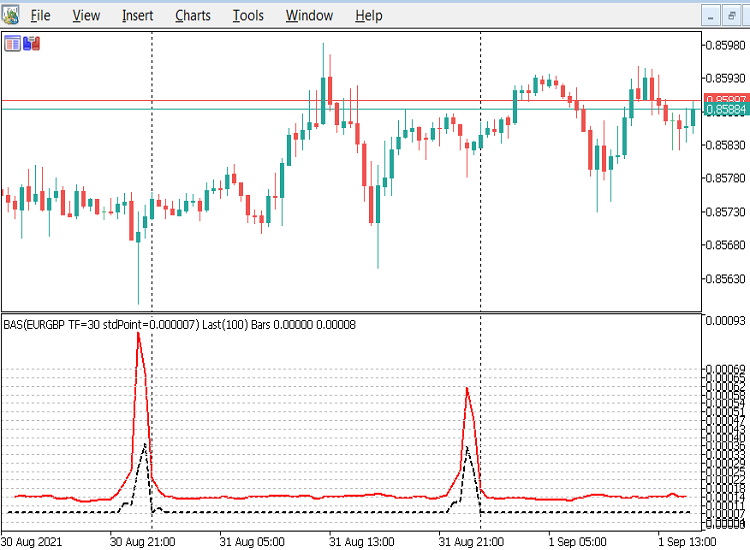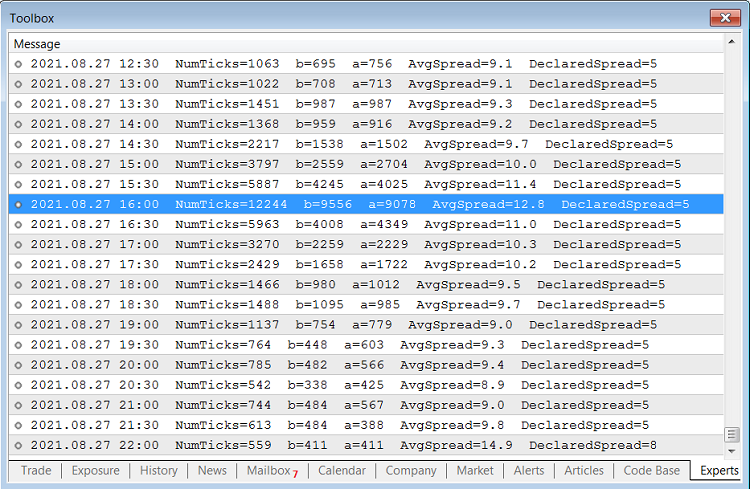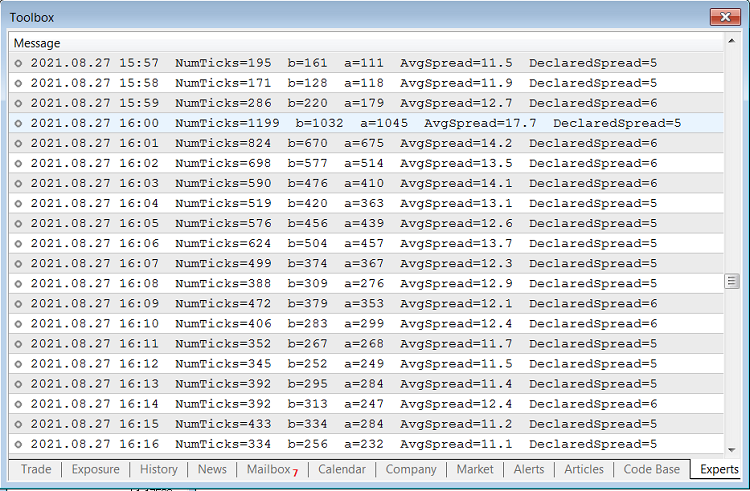### OnCalculate() 代码示例

```//--- Global variables
//--- Set the date formatting for printing to the log
const uint dtFormat=uint(TIME_DATE|TIME_MINUTES);

//+------------------------------------------------------------------+
//| Custom indicator iteration function                              |
//+------------------------------------------------------------------+
int OnCalculate(const int rates_total,
const int prev_calculated,
const datetime &time[],
const double &open[],
const double &high[],
const double &low[],
const double &close[],
const long &tick_volume[],
const long &volume[],
{
//--- Check for no data or Stop flag before we begin
if(_StopFlag || rates_total<2)
{
Alert("Error, StopFlag=", _StopFlag, " #Bars=", rates_total);
return(rates_total);
}

//only do the report at indicator start up or refresh
if(prev_calculated>2)
{
if(prev_calculated==rates_total)
{
int currBar=rates_total-1;
return(rates_total);
}
// else its the start of a new bar so null the ActSpreadBuf
else
{
int currBar=rates_total-1;
return(rates_total);
}
}

static int start=rates_total-numRecentBarsBack;

MqlTick tickBuf[];

int ticks=0;
int bid_tick=0;
int k=0;

for(int i=start; i<rates_total; i++)
{
bid_tick=0;
k=0;

ticks=CopyTicksRange(_Symbol, tickBuf,
COPY_TICKS_INFO, // Only bid and ask changes are required
time[i-1]*1000,  // Start time of previous bar
time[i  ]*1000   // End time of previous bar
);

while(k<ticks)
{

if((tickBuf[k].flags&TICK_FLAG_BID)==TICK_FLAG_BID)
bid_tick++;

k++;
}

// Ensure no divide by zero errors for any missing tick data
if(ticks>0) {
}
else  {
}

if(doPrint)
{
Print(TimeToString(time[i-1], dtFormat),
"  NumTicks="+string(ticks),
"  b="+string(bid_tick),
);
}

}

//don't do stats for incomplete current bar, but can do DeclaredSpread if it has a value

//--- return value of prev_calculated for next call
return(rates_total);
}
```

```ticks=CopyTicksRange(_Symbol, tickBuf,
COPY_TICKS_INFO, // Only bid and ask changes are required
time[i-1]*1000,  // Start time of previous bar
time[i  ]*1000   // End time of previous bar
);
```

### 结束语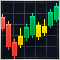探索创建多彩烛条的选项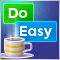DoEasy 函数库中的图形（第八十二部分）：函数库对象重构和图形对象集合DoEasy 函数库中的图形（第八十三部分）：抽象标准图形对象类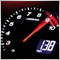更好的程序员（第 04 部分）：如何成为更迅捷的开发人员